# Properties of Quadratic Functions in Standard Form Objectives

• Slides: 14Properties of Quadratic Functions in Standard Form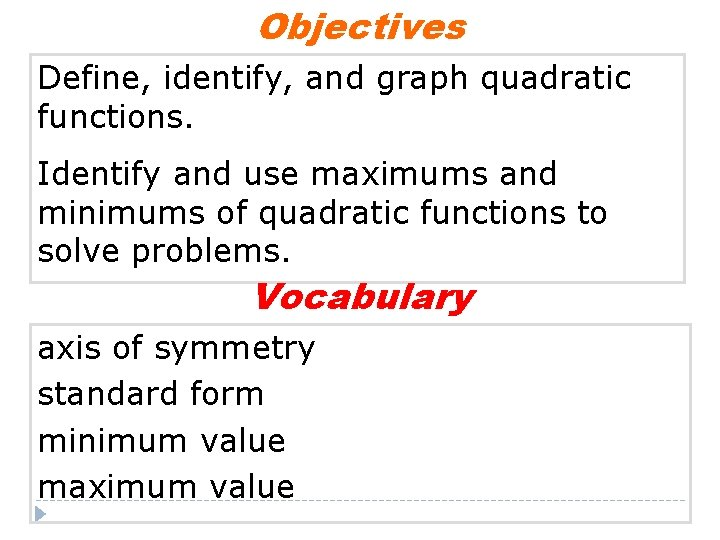Objectives Define, identify, and graph quadratic functions. Identify and use maximums and minimums of quadratic functions to solve problems. Vocabulary axis of symmetry standard form minimum value maximum valueVocabulary �The axis of symmetry is the line through the vertex of a parabola that divides the parabola into two congruent halves. �The standard form of a quadratic function is f(x)= ax 2 + bx + c, where a ≠ 0.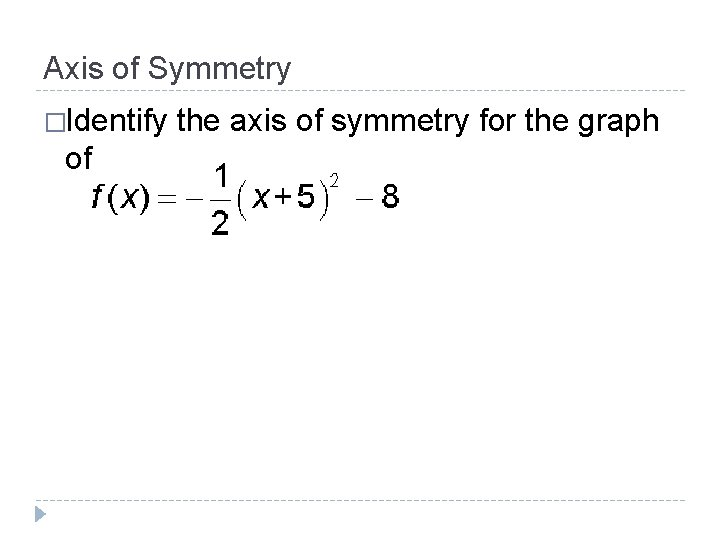Axis of Symmetry �Identify of the axis of symmetry for the graphTransforming vertex form to standard form � 1 st find the components a, h, and k. (vertex form) f(x)= a(x – h)2 + k � Then replace a, b and c with the corresponding values. (standard form)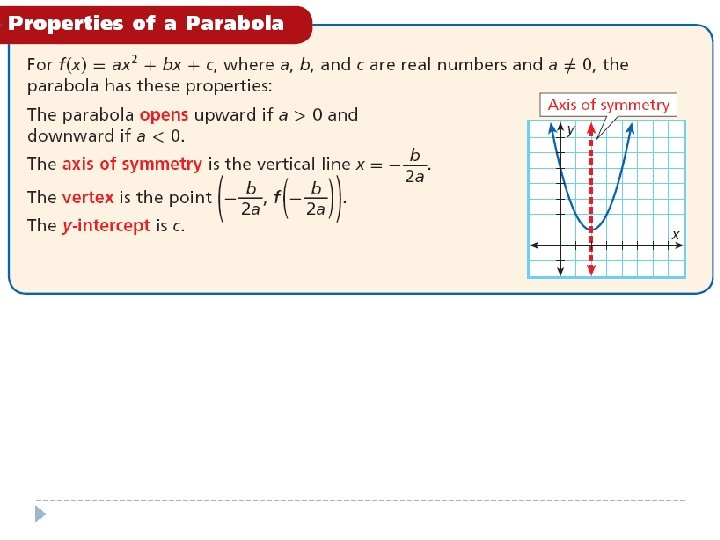Graphing Quadratics in Standard Form Consider the function f(x) = 2 x 2 – 4 x + 5Things you need to graph: Opens: Axis of symmetry: Vertex: y-intercept: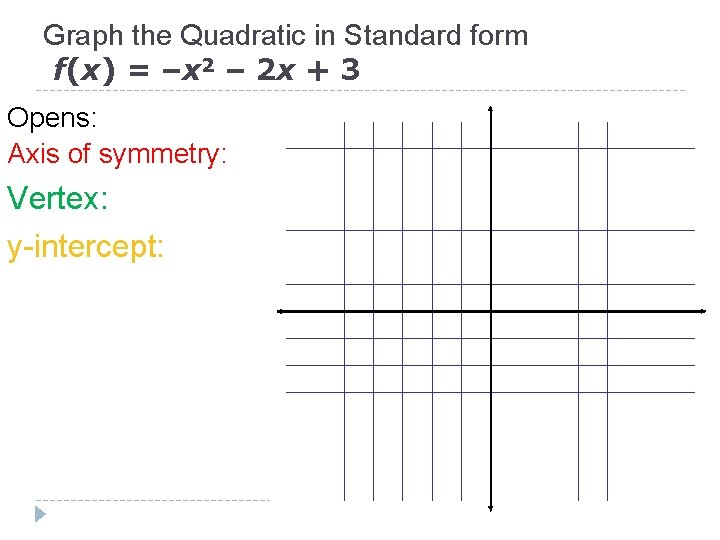Graph the Quadratic in Standard form f(x) = –x 2 – 2 x + 3 Opens: Axis of symmetry: Vertex: y-intercept: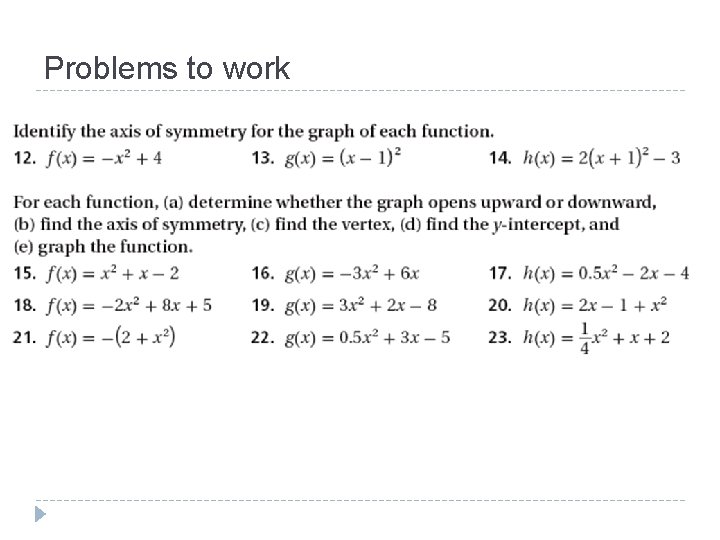Problems to workThe minimum (or maximum) value is the yvalue at the vertex. It is not the ordered pair that represents the vertex.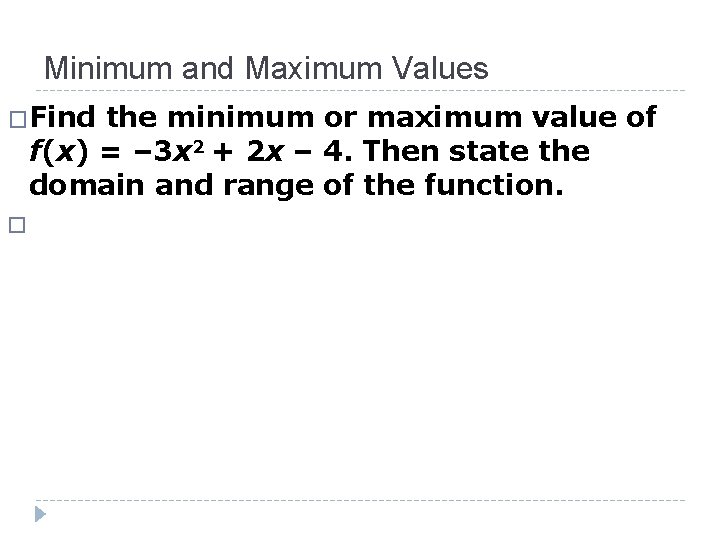Minimum and Maximum Values �Find the minimum or maximum value of f(x) = – 3 x 2 + 2 x – 4. Then state the domain and range of the function. �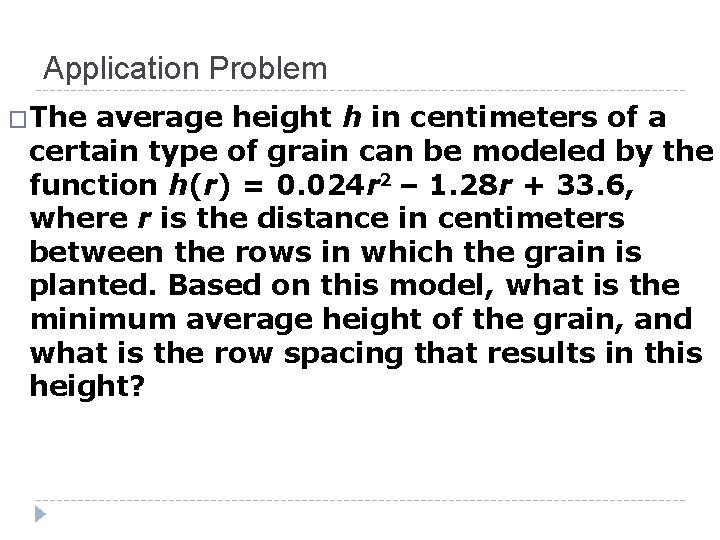Application Problem �The average height h in centimeters of a certain type of grain can be modeled by the function h(r) = 0. 024 r 2 – 1. 28 r + 33. 6, where r is the distance in centimeters between the rows in which the grain is planted. Based on this model, what is the minimum average height of the grain, and what is the row spacing that results in this height?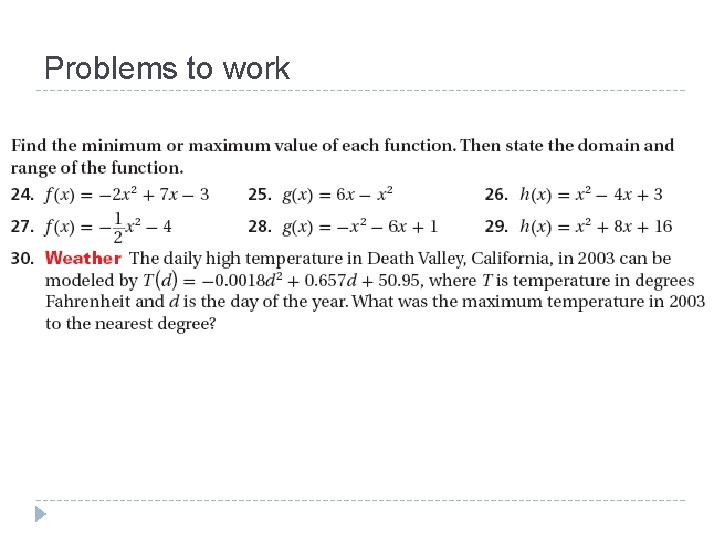Problems to work